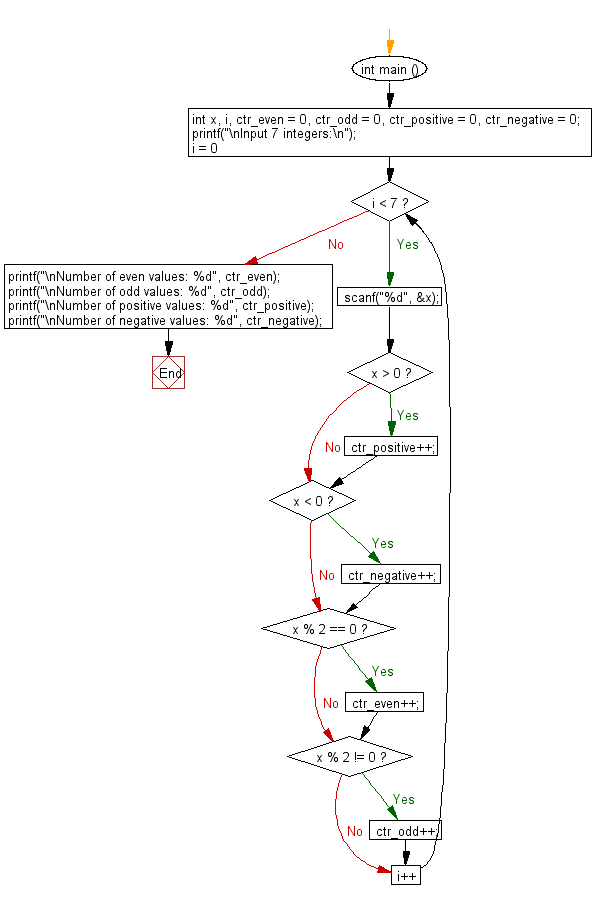﻿ C : Take 7 integers, count even, odd, positive, and negative

# C Exercises: Accept 7 integer values and count the even, odd, positive and negative values

## C Basic Declarations and Expressions: Exercise-106 with Solution

Write a C program that accepts 7 integer values and counts the even, odd, positive and negative values.

Sample Solution:

C Code:

``````#include <stdio.h>

int main ()
{
// Declare variables for input, counters for even, odd, positive, and negative numbers
int x, i, ctr_even = 0, ctr_odd = 0, ctr_positive = 0, ctr_negative = 0;

// Prompt the user to input 7 integers
printf("\nInput 7 integers:\n");

// Loop through 7 times to read the numbers
for (i = 0; i < 7; i++){

scanf("%d", &x);

// Check if the number is positive
if (x > 0){
ctr_positive++;  // Increment positive counter
}

// Check if the number is negative
if (x < 0){
ctr_negative++;  // Increment negative counter
}

// Check if the number is even
if (x % 2 == 0){
ctr_even++;  // Increment even counter
}

// Check if the number is odd
if (x % 2 != 0){
ctr_odd++;  // Increment odd counter
}
}

// Print the counts of even, odd, positive, and negative numbers
printf("\nNumber of even values: %d", ctr_even);
printf("\nNumber of odd values: %d", ctr_odd);
printf("\nNumber of positive values: %d", ctr_positive);
printf("\nNumber of negative values: %d", ctr_negative);
}
``````

Sample Output:

```Input 7 integers:
10
12
15
-15
26
35
17

Number of even values: 3
Number of odd values: 4
Number of positive values: 6
Number of negative values: 1
```

Flowchart:C programming Code Editor:

What is the difficulty level of this exercise?

Test your Programming skills with w3resource's quiz.

﻿